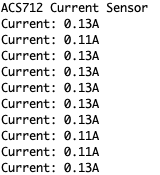# How to use The ACS712 Current Sensor Module

The ACS712 Current Sensor module is a very popular module for measuring the current applied in the circuit due to its capabilities and its price.

In this article, we will talk about how to wire and set up the module for operation with an Arduino Board.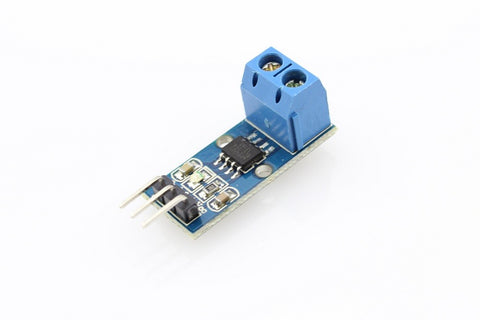## Wiring​

Most ACS712 modules operate at 5V and produce an analog voltage output proportional to the measured current. For this project, we use a power supply (giving output voltage from 0 to 5V), and a resistor to generate current from 0 to 5 A. There are a few versions of the ACS712 Module in the market, but for this article, we will be using ACS712 Current Sensor Module, the one we carry in our shop.

## Wiring Guide​

Connect the pins with male to male jumper wires

• ACS712 GND -- Arduino GND Pin
• ACS712 VCC (5V) -- Arduino 5V Pin
• ACS712 VC -- Arduino A0 Pin
• ACS712 IP- -- Power Supply GND
• ACS712 IP+ -- resistor -- power supply VCC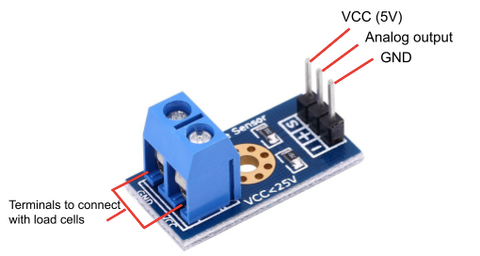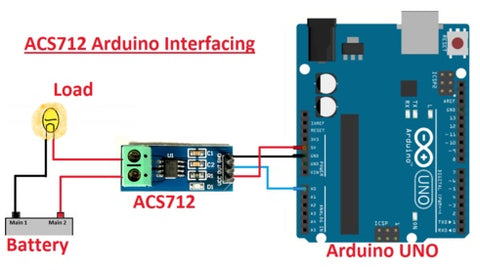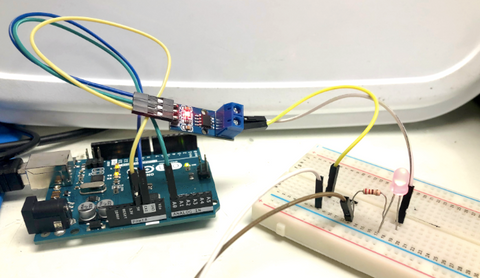## Programming​

Here is the code that will show you the output current in the Serial Monitor. We have set a couple variables in the code. Sensitivity is set to 0.185 according to the module's data sheet. It is based on how sensitive the sensor is to detect current.

In the code, there is a small calculation for measuring the current. First, we assume the VCC from Arduino is giving out 5V to the current sensor module, when there is no current flowing through the terminals, the output voltage of the current sensor is 2.5V. This means you would need to subtract 2.5V from the voltage measured at the analog pin.

Now, in order to calculate the current, divide the value with the sensitivity of the sensor (185mV/A for this sensor).

``float current = (sensor_read - 2500)/sensitivity; //calculate for the current value``

## Full Code:​

``#define VIN A0float sensitivity = 185;void setup() {  Serial.begin(9600);  Serial.println("ACS712 Current Sensor");}void loop() {  float reading = analogRead(A0);  float sensor_read = (reading/1024.0)* 5000; // read the sensor output    float current = abs(((sensor_read - 2500)/sensitivity)); //calculate for the current value  Serial.print("Current: ");  Serial.print(current); // print with 3 decimal places  Serial.println("A");  delay(200); // wait for 2ms for the next calculation}``

## Serial Monitor Output:​

When there is no current passing through in the circuit. Serial Monitor will output "0.00A".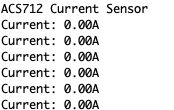When there is current passing through the circuit. It will calculate the current of the load.